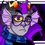# Alternate Derivative Proofs

In this note, I'm going to demonstrate another way to prove the derivative formulas $\frac{d}{dx}f(x)\times g(x)=f'(x)g(x)+g'(x)f(x)$ and $\frac{d}{dx}\frac{f(x)}{g(x)}=\frac{f'(x)g(x)-g'(x)f(x)}{(g(x))^2}$ using logarithmic differentiation. The most commonly taught method is with the definition of a derivative, but this can get ugly and can easily result in a stupid mistake.

First, let's take a look at the derivative of the natural logarithm: $\frac{d}{dx}\ln x.$

If $y=\ln x,$ then $e^y=x.$ Derive both sides with respect to $x.$ $\frac{d}{dy}e^y\frac{dy}{dx}=\frac{d}{dx}x$ $e^y\frac{dy}{dx}=1$ $\frac{dy}{dx}=\frac{1}{e^y}$ $\frac{dy}{dx}=\frac{1}{e^{\ln x}}=\frac{1}{x}$ So that is the derivative of $\ln x.$ If $u$ is a differentiable function in $x,$ then by the chain rule, $\frac{d}{dx}\ln u=\frac{u'}{u}.$ Now we can move to logarithmic differentiation.

Logarithmic differentiation is commonly used for really ugly functions to differentiate. You use it if you are told to derive $\frac{(x-2)^4(x+1)^3}{(x-3)^6},$ for example. If you try to use the chain rule, product rule, and quotient rule for this, you are in for one heck of a ride, and the answer you come up with has a really good chance of being wrong. You can take the natural logarithm of both sides and apply the properties of logarithms and the chain rule to find the answer.

$y=\dfrac{(x-2)^4(x+1)^3}{(x-3)^6}$ $\ln y=\ln \dfrac{(x-2)^4(x+1)^3}{(x-3)^6}$ $\ln y=4\ln(x-2)+3\ln(x+1)-6\ln(x-3)$ $\dfrac{1}{y}\dfrac{dy}{dx}=\dfrac{4}{x-2}+\dfrac{3}{x+1}-\dfrac{6}{x-3}$ $\dfrac{dy}{dx}=y\left(\dfrac{4}{x-2}+\dfrac{3}{x+1}-\dfrac{6}{x-3}\right)$ $\dfrac{dy}{dx}=\left(\dfrac{(x-2)^4(x+1)^3}{(x-3)^6}\right)\left(\dfrac{4}{x-2}+\dfrac{3}{x+1}-\dfrac{6}{x-3}\right)$ This is much easier to evaluate for values of $x.$ If you are actually trying to expand an expression like this, then you are being trolled. A time where you might expand the expression is if you are deriving $\frac{(x+1)^4}{(x-2)^3}.$

So now onto the proofs. $f,$ $g,$ and $F$ are differentiable functions of $x.$

Let's start with the product rule. Let $F=fg$ $F=fg$ $\ln F=\ln fg$ $\ln F=\ln f+\ln g$ $\dfrac{F'}{F}=\dfrac{f'}{f}+\dfrac{g'}{g}$ $\dfrac{F'}{F}=\dfrac{f'g+g'f}{fg}$ $F'=F\left(\dfrac{f'g+g'f}{fg}\right)$ $F'=f'g+g'f$ So this is the proof of the product rule. Now let's move on the quotient rule. Now let $F=\dfrac{f}{g}.$ $F=\dfrac{f}{g}$ $\ln F=\ln\dfrac{f}{g}$ $\ln F=\ln f-\ln g$ $\dfrac{F'}{F}=\dfrac{f'}{f}-\dfrac{g'}{g}$ $\dfrac{F'}{F}=\dfrac{f'g-g'f}{fg}$ $F'=F\left(\dfrac{f'g-g'f}{fg}\right)$ $F'=\left(\dfrac{f}{g}\right)\left(\dfrac{f'g-g'f}{fg}\right)$ $F'=\dfrac{f'g-g'f}{g^2}$ And now the quotient rule has been proven.

In my opinion, this is a lot easier than trying to figure out what you need to add and subtract to the expression found using the definition of a derivative. Hopefully you do too! Thanks for reading this post, and remember to occasionally check #TrevorsTips for tricks to solve problems.Note by Trevor B.
7 years, 6 months ago

This discussion board is a place to discuss our Daily Challenges and the math and science related to those challenges. Explanations are more than just a solution — they should explain the steps and thinking strategies that you used to obtain the solution. Comments should further the discussion of math and science.

When posting on Brilliant:

• Use the emojis to react to an explanation, whether you're congratulating a job well done , or just really confused .
• Ask specific questions about the challenge or the steps in somebody's explanation. Well-posed questions can add a lot to the discussion, but posting "I don't understand!" doesn't help anyone.
• Try to contribute something new to the discussion, whether it is an extension, generalization or other idea related to the challenge.

MarkdownAppears as
*italics* or _italics_ italics
**bold** or __bold__ bold
- bulleted- list
• bulleted
• list
1. numbered2. list
1. numbered
2. list
Note: you must add a full line of space before and after lists for them to show up correctly
paragraph 1paragraph 2

paragraph 1

paragraph 2

[example link](https://brilliant.org)example link
> This is a quote
This is a quote
    # I indented these lines
# 4 spaces, and now they show
# up as a code block.

print "hello world"
# I indented these lines
# 4 spaces, and now they show
# up as a code block.

print "hello world"
MathAppears as
Remember to wrap math in $$ ... $$ or $ ... $ to ensure proper formatting.
2 \times 3 $2 \times 3$
2^{34} $2^{34}$
a_{i-1} $a_{i-1}$
\frac{2}{3} $\frac{2}{3}$
\sqrt{2} $\sqrt{2}$
\sum_{i=1}^3 $\sum_{i=1}^3$
\sin \theta $\sin \theta$
\boxed{123} $\boxed{123}$

Sort by:

Luv u de

- 5 years, 11 months ago

@Trevor B. Can you add these to the Differentiation Rules Wiki pages? Thanks!

Staff - 6 years, 11 months ago

Can anyone think of what else you can use logarithmic differentiation for (in terms of proofs)?

- 7 years, 6 months ago

Something like $x^x$ or even $x^{x^x}$

- 7 years, 6 months ago

right........ it is especially helpful when a function is raised to another function. Here is an interesting example find the derivative of y=x^x^x^x..... up to infinity (try to find the derivative)

- 7 years, 3 months ago

Can you use similar techniques to prove that $\frac{d}{dx}x^n=nx^{n-1}?$

- 7 years, 6 months ago

Let $y = x^n$. Taking the natural logarithm of both sides gives $\ln y = n \ln x$. Differentiating, one has $\frac{d}{dy} \cdot \frac{dy}{dx} \ln y = n \frac{d}{dx} \ln x$ which simplifies to $\frac{dy}{dx}\frac{1}{y} = \frac{n}{x}$. Substituting $y = x^{n}$ back into the equation and rearranging, we find that $\frac{dy}{dx} = nx^{n-1}$, as desired.

- 7 years, 6 months ago

Good job!

- 7 years, 6 months ago

Thanks! It took me a bit too long to format it, though...

- 7 years, 6 months ago

Yup, we can.

Let's say $y = x^n$

$\frac{d}{dx} y = \frac{d}{dx} x^n$

Take the natural log of both sides, and using logarithmic properties we get...

$\frac{d}{dx} lny = \frac{d}{dx} ln x^n = \frac{d}{dx} nln x$

$\frac{1}{y} \frac{dy}{dx} = n\frac{1}{x}$

Multiply both sides by $y$.

$y\frac{1}{y} \frac{dy}{dx} = ny\frac{1}{x}$

And so we get...

$\frac{dy}{dx} = ny\frac{1}{x}$ and remember, $y = x^n$, hence substituting it we get,

$\frac{dy}{dx} = nx^n\frac{1}{x}$

Using exponent properties, I can say...

$\frac{dy}{dx} = nx^n x^{-1}$

$\frac{dy}{dx} = nx^{n-1}$

$y = x^n \Rightarrow \frac{d}{dx} x^n = nx^{n-1}$

I always used to think of interesting ways and they connect beautifully, it's always nice to see something solved in more than one way. ^^

- 7 years, 6 months ago

Good job!

- 7 years, 6 months ago